• +91 9971497814
• info@interviewmaterial.com

# RD Chapter 1- Real Numbers Ex-VSAQS Interview Questions Answers

### Related Subjects

Question 1 : State Euclid’s division lemma.

Euclid’s division lemma:
Let a and b be any two positive integers, then there exist unique integers q and r such that
a = bq + r, 0 ≤ r < b
If b\a, then r = 0, otherwise x. satisfies the stronger inequality 0 < r < b.

Question 2 : State Fundamental Theorem of Arithmetic.

Fundamental Theorem of Arithmetics :
Every composite number can be expressed (factorised) as a product of primes and this factorization is unique except for the order in which the prime factors occur.

Question 3 : Write 98 as product of its prime factors.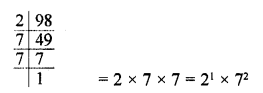Question 4 : Write the exponent of 2 in the prime factorization of 144.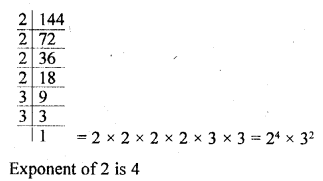Question 5 : Write the sum of the exponents of prime factors in theprime factorization of 98

98 = 2 x 7 x 7 = 21 x72
Sum of exponents = 1 + 2 = 3

Question 6 : If the prime factorization of a natural number n is 23 x 32 x52 x 7, write the number ofconsecutive zeros in n.

n = 23 x 32 x 52 x7
Number of zeros will be 52 x 22 = 102 twozeros

Question 7 : If the product of two numbers is 1080 and their H.C.F. is 30, find their L.C.M.

Product of two numbers = 1080
H.C.F. = 30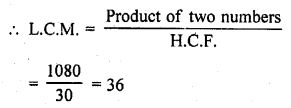Question 8 : Write the condition to be satisfied by q so that a rational number p/q has a terminating decimal expansion. [C.B.S.E. 2008]

In therational number p ,the factorization of denominator q must be in form of 2m x5n where m and n are non-negativeintegers.

Question 9 : Write the condition to be satisfied by q so that a rational number p/q has a non-terminating decimal expansion.

Answer 9 : In the rational number p/q , the factorization of denominator q, is not in the form of 2m x 5n where m and n are non-negative integers.

Question 10 : Complete the missing entries in the following factor tree.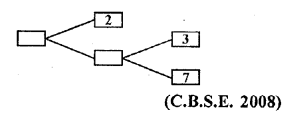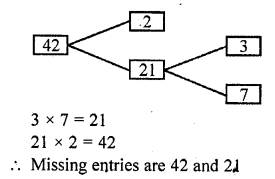Todays Deals### RD Chapter 1- Real Numbers Ex-VSAQS Contributorskrishan

Name:
Email:

# Latest News# 9000 interview questions in different categories